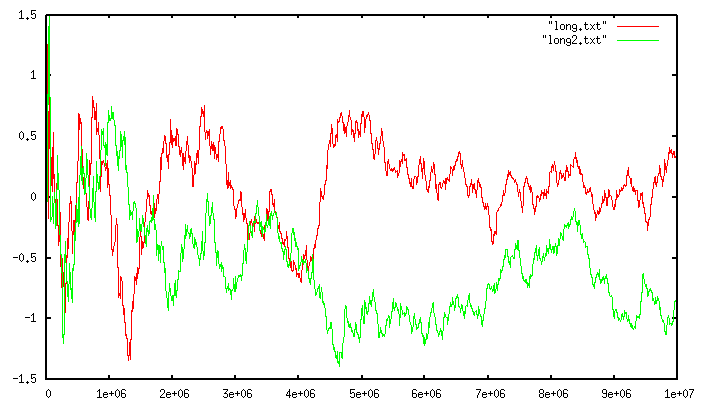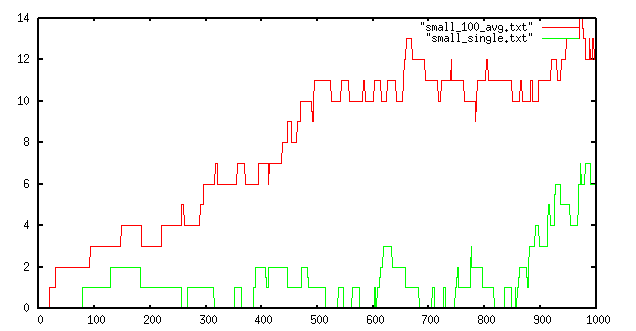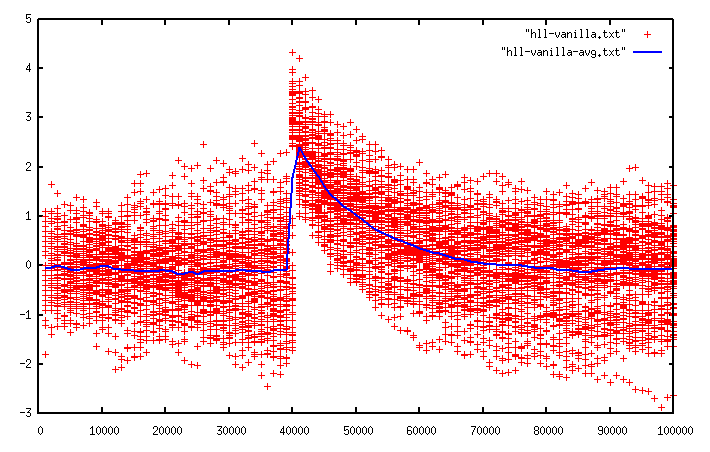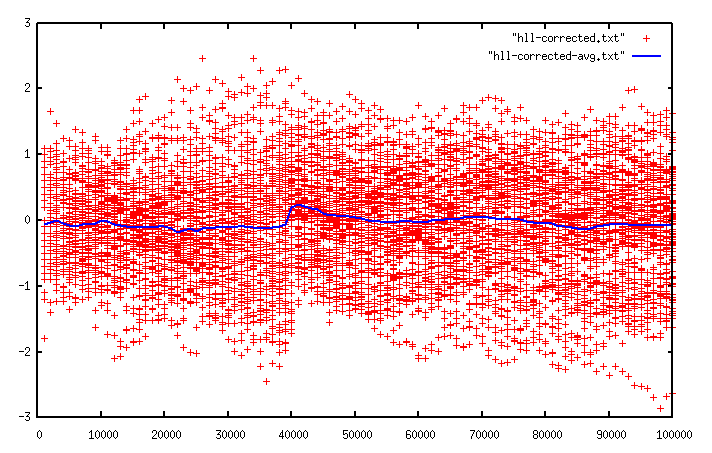# <antirez>

## Redis new data structure: the HyperLogLog

antirez 3529 days ago. 421270 views.
```Generally speaking, I love randomized algorithms, but there is one I love particularly since even after you understand how it works, it still remains magical from a programmer point of view. It accomplishes something that is almost illogical given how little it asks for in terms of time or space. This algorithm is called HyperLogLog, and today it is introduced as a new data structure for Redis.

Counting unique things
===

Usually counting unique things, for example the number of unique IPs that connected today to your web site, or the number of unique searches that your users performed, requires to remember all the unique elements encountered so far, in order to match the next element with the set of already seen elements, and increment a counter only if the new element was never seen before.

This requires an amount of memory proportional to the cardinality (number of items) in the set we are counting, which is, often absolutely prohibitive.

There is a class of algorithms that use randomization in order to provide an approximation of the number of unique elements in a set using just a constant, and small, amount of memory. The best of such algorithms currently known is called HyperLogLog, and is due to Philippe Flajolet.

HyperLogLog is remarkable as it provides a very good approximation of the cardinality of a set even using a very small amount of memory. In the Redis implementation it only uses 12kbytes per key to count with a standard error of 0.81%, and there is no limit to the number of items you can count, unless you approach 2^64 items (which seems quite unlikely).

The algorithm is documented in the original paper , and its practical implementation and variants were covered in depth by a 2013 paper from Google .

 http://algo.inria.fr/flajolet/Publications/FlFuGaMe07.pdf

How it works?
===

 http://blog.aggregateknowledge.com/2012/10/25/sketch-of-the-day-hyperloglog-cornerstone-of-a-big-data-infrastructure/

Here I’ll cover only the basic idea using a very clever example found at . Imagine you tell me you spent your day flipping a coin, counting how many times you encountered a non interrupted run of heads. If you tell me that the maximum run was of 3 heads, I can imagine that you did not really flipped the coin a lot of times. If instead your longest run was 13, you probably spent a lot of time flipping the coin.

However if you get lucky and the first time you get 10 heads, an event that is unlikely but possible, and then stop flipping your coin, I’ll provide you a very wrong approximation of the time you spent flipping the coin. So I may ask you to repeat the experiment, but this time using 10 coins, and 10 different piece of papers, one per coin, where you record the longest run of heads. This time since I can observe more data, my estimation will be better.

Long story short this is what HyperLogLog does: it hashes every new element you observe. Part of the hash is used to index a register (the coin+paper pair, in our previous example. Basically we are splitting the original set into m subsets). The other part of the hash is used to count the longest run of leading zeroes in the hash (our run of heads). The probability of a run of N+1 zeroes is half the probability of a run of length N, so observing the value of the different registers, that are set to the maximum run of zeroes observed so far for a given subset, HyperLogLog is able to provide a very good approximated cardinality.

The Redis implementation
===

The standard error of HyperLogLog is 1.04/sqrt(m), where “m” is the number of registers used.
Redis uses 16384 registers, so the standard error is 0.81%.

Since the hash function used in the Redis implementation has a 64 bit output, and we use 14 bits of the hash output in order to address our 16k registers, we are left with 50 bits, so the longest run of zeroes we can encounter will fit a 6 bit register. This is why a Redis HyperLogLog value only uses 12k bytes for 16k registers.

Because of the use of a 64 bit output function, which is one of the modifications of the algorithm that Google presented in , there are no practical limits to the cardinality of the sets we can count. Moreover it is worth to note that the error for very small cardinalities tend to be very small. The following graph shows a run of the algorithm against two different large sets. The cardinality of the set is shown in the x axis, while the relative error (in percentage) in the y axis.The red and green lines are two different runs with two totally unrelated sets. It shows how the error is consistent as the cardinality increases. However for much smaller cardinalities, you can enjoy a much smaller error:The green line shows the error of a single run up to cardinality 100, while the red line is the maximum error found in 100 runs. Up to a cardinality of a few hundreds the algorithm is very likely to make a very small error or to provide the exact answer. This is very valuable when the computed value is shown to an user that can visually match if the answer is correct.

The source code of the Redis implementation is available at Github:

https://github.com/antirez/redis/blob/unstable/src/hyperloglog.c

The API
===

From the point of view of Redis an HyperLogLog is just a string, that happens to be exactly 12k + 8 bytes in length
(12296 bytes to be precise). All the HyperLogLog commands will happily run if called with a String value exactly of this size, or will report an error. However all the calls are safe whatever is stored in the string: you can store garbage and still ask for an estimation of the cardinality. In no case this will make the server crash.

Also everything in the representation is endian neutral and is not affected by the processor word size, so a 32 bit big endian processor can read the HLL of a 64 bit little endian processor.

The fact that HyperLogLogs are strings avoided the introduction of an actual type at RDB level. This allows the work to be back ported into Redis 2.8 in the next days, so you’ll be able to use HyperLogLogs ASAP. Moreover the format is automatically serialized, and can be retrieved and restored easily.

The API is constituted of three new commands:

PFADD var element element … element
PFCOUNT var
PFMERGE dst src src src … src

The commands prefix is “PF” in honor of Philippe Flajolet .

 http://en.wikipedia.org/wiki/Philippe_Flajolet

PFADD adds elements to the HLL stored at “var”. If the variable does not exist, an empty HLL is automatically created as it happens always with Redis API calls. The command is variadic, so allows for very aggressive pipelining and mass insertion.

The command returns 1 if the underlying HyperLogLog was modified, otherwise 0 is returned.
This is interesting for the user since as we add elements the probability of an element actually modifying some register decreases. The fact that the API is able to provide hints about the fact that a new cardinality is available allows for programs that continuously add elements and retrieve the approximated cardinality only when a new one is available.

PFCOUNT returns the estimated cardinality, which is zero if the key does not exist.

Finallly PFMERGE can merge N different HLL values into one. The resulting HLL will report an estimated cardinality that is the cardinality of the union of the different sets that we counted with the different HLL values.
This seems magical but works because HLL while randomized is fully deterministic, so PFMERGE just takes, for every register, the maximum value available across the N HLL values. A given element hashes to the same register with the same run of zeroes always, so the merge performed in this way will only add the count of the elements that are not common to the different HLLs.

As you can see HyperLogLog is fully parallelizable, since it is possible to split a set into N subsets counted independently to later merge the values and obtain the total cardinality approximation. The fact that HLLs in Redis are just strings helps to move HLL values across instances.

First make it correct, then make it fast
===

Redis HHLs are composed of 16k registers packed into 6 bit integers. This creates several performance issues that must be solved in order to provide an API of commands that can be called without thinking too much.

One problem is that accessing to registers require accessing multiple bytes, shifting, and masking in order to retrieve the correct 6 bit value. This is not a big problem for PFADD that only touches a register for every element, but PFCOUNT needs to perform a computation using all the 16k registers, so if there are non trivial constant times to access every single register, the command risks to be slow. Moreover, while accessing the registers, we need to compute the sum of pow(2,-register) which involves floating point math.

One may feel the temptation of using full bytes instead of 6 bit integers in order to speedup the computation, however this would be a shame since every HLL would use 16k instead of 12k that is a non trivial difference, so this route was discarded at the beginning. The command was optimized for a speedup of about 3 times compared to the initial implementation by doing the following changes:

* For m=16k which is the Redis default (the implementation is more generic and could theoretically work with different values) the implementation selects a fast-path with unrolled loops accessing 16 register at every time. The registers are accessed using fixed offsets / shifts / masks (via some pointer that is incremented 12 bytes at the next iteration).
* The floating point computation was modified in order to allow for multiple operations to be performed in parallel when possible. This was just a matter of adding parens. Floating point math is not commutative, but in this case there was no loss of precision.
* The pow(2,-register) term was precomputed in a lookup table.

With the 3x speedup provided by the above changes the command was able to perform about 60k calls per second in a fast hardware. However this is still far from the hundreds thousands calls possible with commands that are, from the user point of view, conceptually similar, like SCARD.

Instead of optimizing the computation of the approximated cardinality further, there was a simpler solution. Basically the output of the algorithm only changes if some register changes. However as already observed above, most of the PFADD calls don’t result in any register changed. This basically means that it is possible to cache the last output and recompute it only if some register changes.

So our data structure has an additional tail of 8 bytes representing a 64bit unsigned integer in little endian format. If the most significant bit is set, then the precomputed value is stale and requires to be recomputed, otherwise PFCOUNT can use it as it is. PFADD just turns on the “invalid cache” bit when some register is modified.

After this change even trying to add elements at maximum speed using a pipeline of 32 elements with 50 simultaneous clients, PFCOUNT was able to perform as well as any other O(1) command with very small constant times.

Bias correction using polynomial regression
===

The HLL algorithm, in order to be practical, must work equally well in any cardinality range. Unfortunately the raw estimation performed by the algorithm is not very good for cardinalities less than m*2.5 (around 40000 elements for m=16384) since in this range the algorithm outputs biased or even results with larger errors depending on the exact range.

The original HLL paper  suggests switching to Linear Counting  when the raw cardinality estimated by the first part of the HLL algorithm is less than m*2.5.

 http://dblab.kaist.ac.kr/Publication/pdf/ACM90_TODS_v15n2.pdf

Linear counting is a different cardinality estimator that uses a simple concept. We have a bitmap of N bits. Every time a new element must be counted, it is hashed, and the hash is used in order to index a random bit inside the bitmap, that is turned to 1. The number of unset bits in the bitmap gives an idea of how many elements we added so far using the following formula:

cardinality = m*log(m/ez);

Where ‘ez’ is the number of zero bits and m is the total number of bits in the bitmap.

Linear counting does not work well for large cardinalities compared to HyperLogLog, but works very well for small cardinalities. Since the HLL registers as a side effect also work as a linear counting bitmap, counting the number of zero registers it is possible to apply linear counting for the range where HLL does not perform well. Note that this is possible because when we update the registers, we don’t really use the longest run of zeroes, but the longest run of zeroes plus one. This means that if an element is added and it is addressing a register that was never addressed, the register will turn from 0 to a different value (at least 1).

The problem with linear counting is that as the cardinality gets bigger, its output error gets larger, so we need to switch to HLL ASAP. However when we switch at 2.5m, HLL is still biased. In the following image the same cardinality was tested with 1000 different sets, and the error of each run is reported as a point:The blu line is the average of the error. As you can see before a cardinality of 40k, where linear counting is used, the more we go towards greater cardinalities, the more the points “beam” gets larger (bigger errors). When we switch to HLL raw estimate the error is smaller, but there is a bias: the algorithm overestimates the cardinality in the range 40k-80k.

Google engineers studied this problem extensively  in order to correct the bias. Their solution was to create an empirical table of cardinality values and the corresponding biases. Their modified algorithm uses the table and interpolation in order to get the bias in a given range, and correct accordingly.

I used a different approach: you can see that the bias is not random but looks like a very smooth curve, so I calculated a few cardinality-bias samples and performed polynomial regression in order to find a polynomial approximating the curve.

Currently I’m using a four order polynomial to correct in the range 40960-72000, and the following is the result after the bias correction:While there is still some bias at the switching point between the two algorithms, the result is quite satisfying compared to the vanilla HLL algorithm, however it is probably possible to use a curve that fits better the bias curve. I had no time to investigate this further.

It is worth to note that during my investigations I found that, when no bias correction is used, and at least for m=16384, the best value to switch from linear counting to raw HLL estimate is actually near 3 and not 2.5 as mentioned in , since a value of 3 both improves bias and error. Values larger than 3 will improve the bias (a value of 4 completely corrects it) but will have bad effects on the error.

The original HLL algorithm also corrects for values towards 2^32  since once we approach very large values collisions in the hash function starts to be an issue. We don’t need such correction since we use a 64 bit hash function and 6 bits counters, which is one of the modifications proposed by Google engineers  and adopted by the Redis implementation.

Future work
===

Intuitively it seems like it is possible to improve the error of the algorithm output when linear counting is used by exploiting the additional informations we have. In the standard linear counting algorithm the registers are just 1 bit wide, so we have only two informations: if an element so far hashed to this bit or not. Still the HLL algorithm as proposed initially  and as modified at Google , when reverting to linear counting still only use the number of zero registers as the input of the algorithm. It is possible that also using the information stored in the registers could improve the output.

For example in standard linear counting, assuming we have 10 bits, I may add 5 elements that all happen to address the same bit. This is an odd case that the algorithm has no way to correct, and the estimation provided will likely be smaller than the actual cardinality. However in the linear counting algorithm used by HLL in a similar situation we may found that the value at the only register set is an hint about multiple elements colliding there, allowing a correction of the output.

Conclusion
===

HyperLogLog is an amazing data structure. My hope is that the Redis implementation, that will be available in a stable release in a matter of days (Redis 2.8.9 will include it), will provide this tool in a ready to use form to many programmers.

The HN post is here: https://news.ycombinator.com/item?id=7506774```